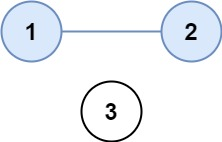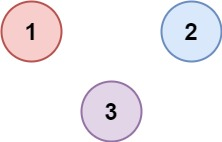### LeetCode• ㊗️
• 大家
• offer
• 多多！

## Problem

There are n cities. Some of them are connected, while some are not. If city a is connected directly with city b, and city b is connected directly with city c, then city a is connected indirectly with city c.

A province is a group of directly or indirectly connected cities and no other cities outside of the group.

You are given an n x n matrix isConnected where isConnected[i][j] = 1 if the ith city and the jth city are directly connected, and isConnected[i][j] = 0 otherwise.

Return the total number of provinces.

Example 1:Input: isConnected = [[1,1,0],[1,1,0],[0,0,1]]
Output: 2


Example 2:Input: isConnected = [[1,0,0],[0,1,0],[0,0,1]]
Output: 3


Constraints:

• 1 <= n <= 200
• n == isConnected.length
• n == isConnected[i].length
• isConnected[i][j] is 1 or 0.
• isConnected[i][i] == 1
• isConnected[i][j] == isConnected[j][i]

## Code

DFS

class Solution {
public int findCircleNum(int[][] isConnected) {
int count = 0;

boolean[] visited = new boolean[isConnected.length];

for (int i = 0; i < isConnected.length; i++) {
if (!visited[i]) {
helper(isConnected, visited, i);
count++;
}
}

return count;
}

private void helper(int[][] matrix, boolean[] visited, int curr) {
for (int i = 0; i < matrix.length; i++) {
if (matrix[curr][i] == 1 && !visited[i]) {
visited[i] = true;
helper(matrix, visited, i);
}
}
}
}


BFS

class Solution {
public int findCircleNum(int[][] isConnected) {
int count = 0;

boolean[] visited = new boolean[isConnected.length];

for (int i = 0; i < isConnected.length; i++) {
if (!visited[i]) {
queue.offer(i);

while (!queue.isEmpty()) {
int curr = queue.poll();
visited[curr] = true;

for (int j = 0; j < isConnected.length; j++) {
if (isConnected[curr][j] == 1 && !visited[j])
queue.offer(j);
}
}

count++;
}
}

return count;
}
}

class Solution {
public class UnionFind {
int[] parents;
int groupNum = 0;

public UnionFind(int n) {
parents = new int[n];

for (int i = 0; i < n; i++) {
parents[i] = i;
}

groupNum = n;
}

public boolean union(int x, int y) {
int xParent = find(x);
int yParent = find(y);

if (xParent == yParent) return false;

groupNum--;
parents[yParent] = xParent;

return true;
}

public int find(int node) {
while (node != parents[node]) {
int parentNode = parents[parents[node]];

// parents[node] = parentNode;
node = parentNode;
}

return node;
}

public int getGroupNum() {
return groupNum;
}
}

public int findCircleNum(int[][] isConnected) {
int n = isConnected.length;
UnionFind unionFind = new UnionFind(n);

for (int i = 0; i < n; i++) {
for (int j = i + 1; j < n; j++) {
if (isConnected[i][j] == 1) {
unionFind.union(i, j);
}
}
}

return unionFind.getGroupNum();
}
}